## Practice Numerical Reasoning

Practice Now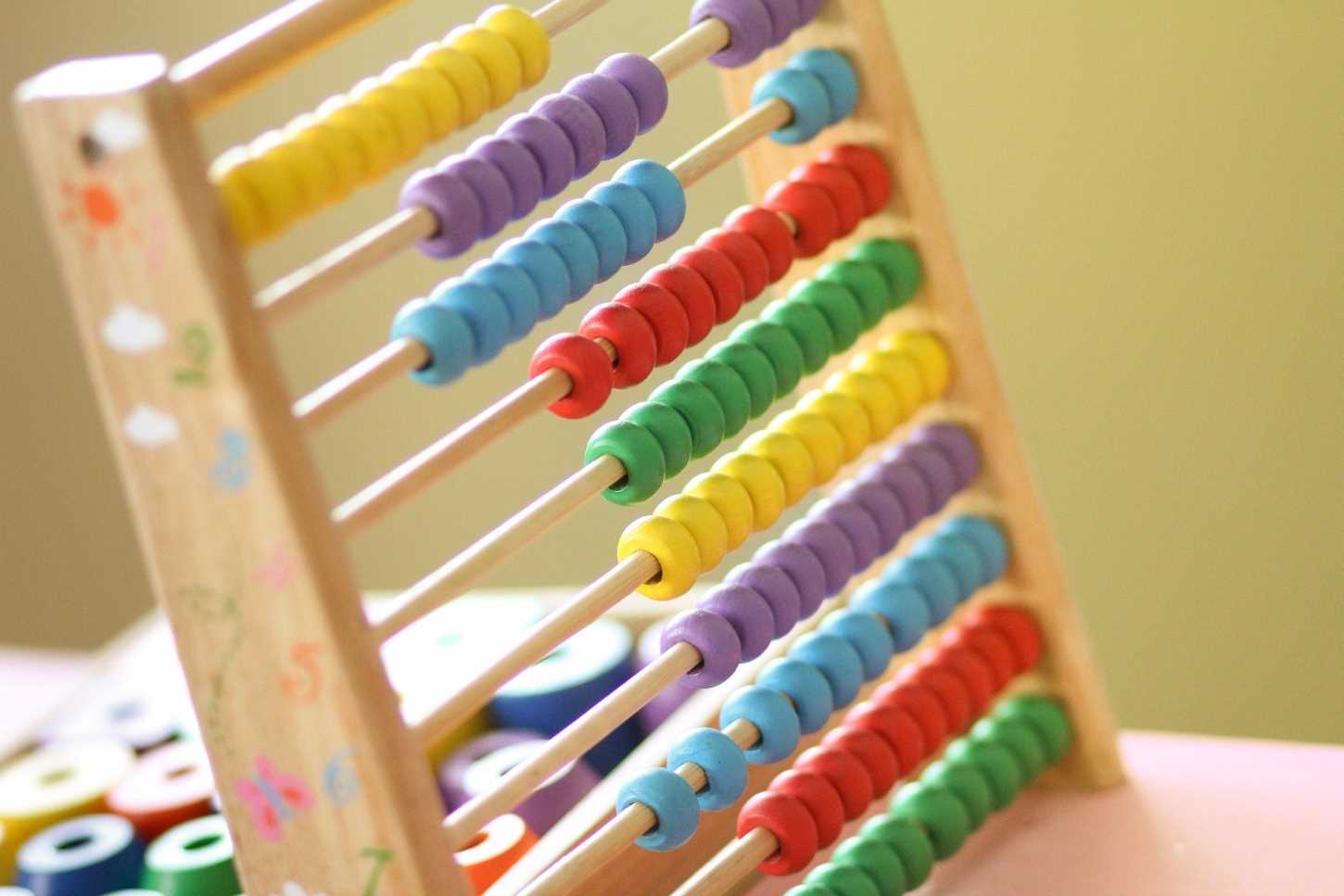# How to Solve Questions About Ratios

Updated 19 January 2021

0
shares

## What Is a Ratio?

A ratio is a mathematical term that is used to compare the size of one number to the size of another number. It is commonly used in both mathematics and in professional environments.

Here are some everyday examples of times when you could use ratios:

• When you convert your Pounds to Dollars or Euros when you go on holiday
• When you calculate your winnings on a bet
• When you work out how many bottles of beer you need for a party
• When you share a packet of sweets fairly among your friends
• When you calculate how much tax you must pay on your income

Ratios are usually used to compare two numbers, though they can also be used to compare multiple quantities.

Ratios are often included in numerical reasoning tests, where they can be presented in a number of different ways. It is therefore important that you are able to recognize and manipulate ratios however they are presented.

## Different Ways of Presenting Ratios

Ratios are usually shown as two or more numbers separated with a colon, for example, 8:5 or 1:4 or 3:2:1.

However, they can also be shown in a number of other ways; the three examples below are all different expressions of the same ratio.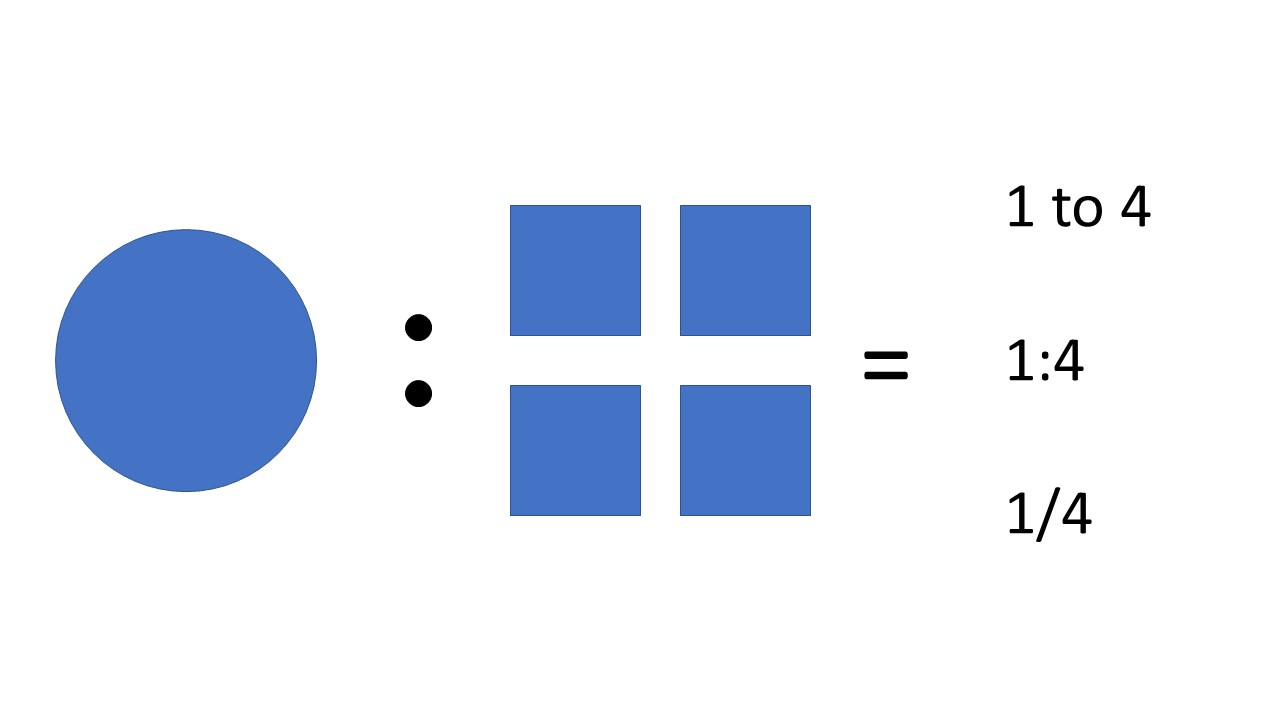## Scaling a Ratio

One of the reasons that ratios are useful is that they enable us to scale amounts. This means increasing or decreasing the amount of something. This is particularly handy for things like scale models or maps, where really big numbers can be converted to much smaller representations that are still accurate.

Scaling is also helpful for increasing or decreasing the amount of ingredients in a recipe or chemical reaction. Ratios can be scaled up or down by multiplying both parts of the ratio by the same number.

For example: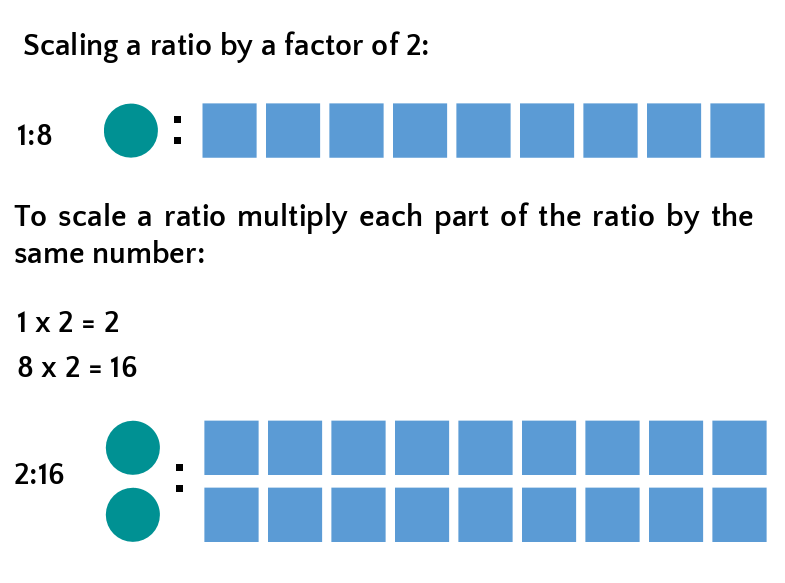There are six practice questions below. Should you need further practice afterwards, we recommend the numerical reasoning packages available from JobTestPrep. These tests include ratio questions, with full explanations for all answers.

### Question 1: Scaling Ratios

Olivia wants to make pancakes for nine friends, but her recipe only makes enough pancakes for three friends (the recipe is shown below). What quantity of the ingredients will she need to use?

Pancake recipe (serves 3)

• 100g flour
• 300ml milk
• 2 large eggs

To solve this question, you must first identify the ratio. This is a three-part ratio, whereby:

• 100g flour
• 300ml milk
• 2 large eggs

= 100:300:2

Next, you need to work out how much you need to scale the recipe by.

This recipe is for three people, but Olivia needs a recipe for nine.

As 9/3 = 3, the ratio needs to be scaled by three (this is sometimes expressed as by a factor of 3).

You then need to multiply each part of the ratio by 3:

• 100 x 3 = 300
• 300 x 3 = 900
• 2 x 3 = 6

Therefore, to make enough pancakes for nine people, Olivia will need to use 300g flour, 900ml milk and 6 large eggs.

## Reducing a Ratio

Sometimes ratios are not presented in their simplest form, which makes them harder to manipulate.

For example, if a farmer has seven chickens and together they lay 56 eggs every day, this would be represented by the ratio 7:56 (or presented as a fraction this would be shown as 7/56).

Reducing a ratio means converting the ratio to its simplest form, and this makes it easier to use. This is done by dividing both numbers in of the ratio by the largest number that they can both be divided by (this is just the same as reducing a fraction to its simplest form).

So, for example: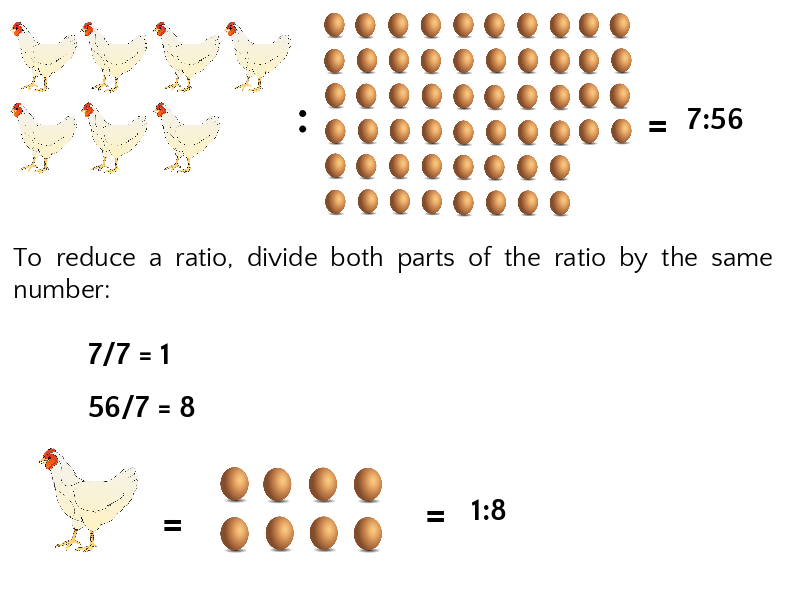Therefore, the reduced ratio is 1:8, which tells us that each chicken lays eight eggs. This is far easier to use. For example, if the farmer needed 96 eggs per day, he could use the ratio to work out how many chickens he would need for this to be possible.

We know from the ratio that each chicken lays 8 eggs, therefore, if the farmer wants 96 eggs he must divide 96 by 8 to calculate the number of chickens required:

96/8 = 12 chickens

Similarly, if one of the farmer’s chickens stopped laying eggs, he could use the ratio to work out how much that would reduce his total number of eggs to; for example, 56 – 8 = 48.

### Question 2: Reducing Ratios

Ella has 18 pigeons and they eat 54kg of grain per week. Jayden has 22 pigeons and they eat 88kg or grain per week. Who has the greediest pigeons?

To solve this question, you must first identify, then simplify the two ratios:

• Ella’s ratio = 18:54, simplify this by dividing both numbers by 18, which gives a ratio of 1:3

• Jayden’s ratio = 22:88, simplify this by dividing both numbers by 22, which gives a ratio of 1:4

This tells us that Ella’s pigeons each eat 3kg of grain per week, whilst Jayden’s eat 4kg of grain per week. Therefore, Jayden’s pigeons are greedier.

## Finding Unknown Quantities From Existing Equivalent Ratios

One of the ways that ratios are particularly useful is that they enable us to work out new and unknown quantities based on an existing (known) ratio. There are a couple of ways of solving this type of problem. The first is to use cross-multiplication.

For example, there are four rings and seven bracelets in the jewellery box. If this ratio were maintained, how many necklaces would there be if there were 28 bracelets?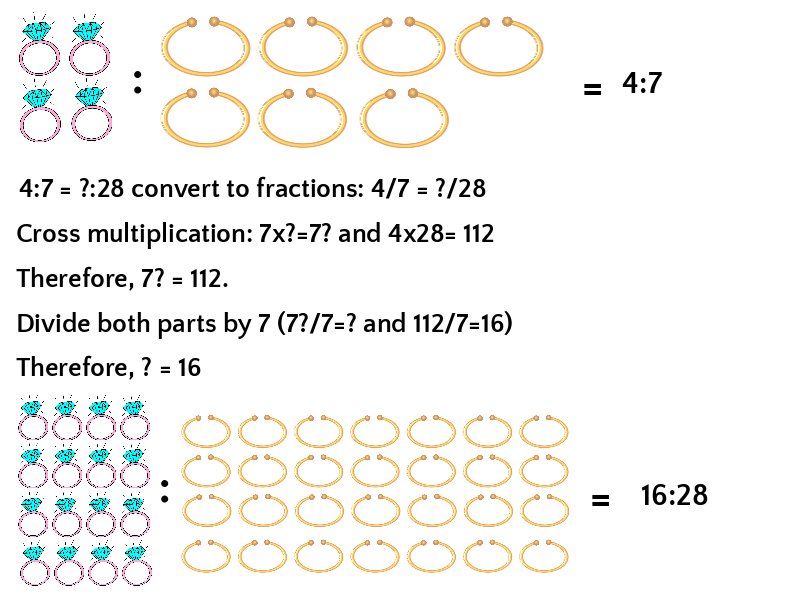Another method is to calculate the factor (size) of the increase in number of bracelets (28/7 = 4) and to then multiply the number of rings by that same number (4 x 4 = 16).

### Question 3: Finding Unknown Quantities From Existing Equivalent Ratios

Gabriel and Mandeep are getting married. They have calculated that they will need 35 bottles of wine for their 70 guests. Suddenly they find out that another 20 guests are planning to attend. How much wine do they need in total?

First, you need to work out the ratio of wine to guests they used = 35 wine:70 guests.

Then simplify this so 1 wine:2 guests (or 0.5 bottle wine per guest).

They now have 90 guests attending (70 + the extra 20 = 90) so you need to multiply 90 by 0.5 = 45 bottles of wine in total.

Watch out for the wording in this sort of question, which can sometimes ask for the total required, and sometimes the extra required.

## Common Mistakes and Things to Look Out For

• Make sure you are reading the ratio the right way. For example, a ratio of 2 pink to 6 red should be expressed 2:6 not 6:2. The first item in the sentence comes first.

• Be careful with reading the wording. For example, people often make mistakes with questions such as “Bob has eight pigs and four cows. Calculate the ratio of pigs to animals.” It can be tempting to say the ratio is 8:4, but that would be incorrect because the question asks for the ratio of pigs to animals. Therefore you need to calculate the total number of animals (8 + 4 = 12), so the correct ratio is, therefore, 8:12 (or 2:3).

• Don’t be put off by units or decimals. The principles remain the same whether they apply to whole numbers, fractions, £ or m2, but do ensure you note the units in your calculations, and where possible convert them to the same units. For example, if you have a ratio of 500g to 0.75kg, then convert both sides to either grams or kilos.

### Question 4: Scaling Ratios

Otto knows that his car requires 27.5 litres of fuel to drive 110 miles. He wants to drive from London to Edinburgh, a distance of 405 miles. How much fuel will he need?

• To calculate this you first need to work out the ratio of fuel to miles driven = 27.5:110

• The next step is to simplify this ratio by dividing both halves of the ratio by 27.5 to calculate how much fuel is required to drive one mile: 27.5/27.5 = 1, 110/27.5 = 4 the ratio is therefore 1:4. One litre of fuel is required to drive four miles.

• Next you need to scale the ratio. 405/4=101.25. So Otto needs 101.25 litres of fuel to drive his car 405 miles.

### Question 5: Reducing Ratios

Hugo and Claudia share 600g chocolate using the ratio 4:2. How much chocolate does Claudia get?

To solve this question, you must first add together the two halves of the ratio i.e. 4 + 2 = 6.

Then you need to divide the total amount using that number i.e. 600/6 = 100.

To work out how much each person gets, you then multiply their share by 100.

So Hugo gets 400g and Claudia gets 200g.

### Question 6: Scaling Ratios

Caleb wants to paint his house. He checks the instructions on the paint tin and finds that one tin of paint will cover 5m2. The total wall area Caleb needs to paint is 40m2. How many tins of paint will he need to buy?

• First, you need to identify the ratio of paint tin to wall area. This is 1:5m2
• Next, you need to work out how much you need to scale the ratio by. To do this you need to divide 40m2 by 5m2 (because Caleb has a total wall area of 40m2 but we only know the paint ratio of 5m2, therefore we need to know how much bigger than 5m2 40m2 is).
• 40/5 = 8; we, therefore, need to scale the ratio by 8.
• To do this you need to multiply each part of the ratio by 8: 1 x 8 = 8, 5 x 8 = 40
• Use this to create your new ratio = 8:40m2. This tells us that Caleb will need 8 tins of paint.

If you would like to practise more ratio-based questions, WikiJob has a psychometric tests app, available in both iTunes and Google Play. All tests include a timer and worked solutions at the end. Give it a try!

## Want to Practice More?

Aptitude Tests Platform
Fast Learning Results
Get the Job You Want